Basic Electronics

# Operational amplifier OP AMP Introduction, Symbol and Characteristics

## Operational amplifier OP-AMP:

The term operational amplifier is usually used for amplifiers that have extremely high gain, extremely high input impedance, and very low output impedance.  It is a directly coupled negative field back voltage amplifier with a differential input (i.e. there are two input terminals on its input, none of which has been grounded). As it is directly coupled (i.e. coupling capacitors are not used along it to block DC), therefore this can amplify signals, the frequency range of which is between 0 Hertz to 1 MHZ. In simple words, an operational amplifier is a high gain amplifier circuit, which consists of two high impedance input terminals and one low impedance output. Identification of inputs is done via inverting and non-inverting inputs. Its basic circuit consists of a differential amplifier input stage and an emitter follower output stage. Remember that an operational amplifier is basically a modified form of a differential amplifier wherein its peculiar characteristics and performance is improved by including extra circuitry. As this amplifier has been designed in order to perform mathematical operations in analogue computers (i.e. addition, subtraction, multiplication, differentiation, and integration, etc.), that’s the reason it is known as an operational amplifier (or simply OP-AMP). In other words, an amplifier, which performs mathematical operations in analogue computers, is called an operational amplifier. This amplifier is widely used in every branch of electronics, particularly in electronic control systems.

In the beginning, operational amplifiers were manufactured through discrete devices (these devices were fabricated with vacuum tubes and worked on high voltages). However, the invention of integrated circuits made their fabrication quite easy. An operational amplifier is a device that works as an important building block in the design of different electronics circuits. These days, such circuits are made along with various internal arrangements of linear integrated circuits. They are very reliable, cheap, and work on relatively low DC voltages. They can successfully be operated between 0 to 1 MHZ. In fact, an operational amplifier is not a discrete or individual device. Rather it is such a complicated circuit that is composed of several components (i.e. it has about 20 -25 devices along with a transistor, diode, and resistor). An operational amplifier IC may contain 2 dozen transistors, one dozen resistors, and one or two capacitors, however, practically it is considered a single device. It is used in a closed case/ shell, along which only external connections are furnished for the user. As a majority of operational amplifiers are IC type these days, therefore, the study of their input and output characteristics is very important.

Although an operational amplifier is a sort of complete amplifier, however, it is designed in such a manner that external components (resistors, capacitors etc.) can also attached along with its terminals, so that its external characteristics (e.g. amplifier voltage gain, input and output impedance and frequency response, etc.) could be changed. Thus, setting up this kind of amplifier for some particular purpose is relatively easy. And due to its versatility, its usage has gained tremendous popularity in the industry.

Sometimes, the output of an operational amplifier is connected with any one of its inputs directly or indirectly, this is called feedback. When feedback is provided to an operational amplifier, we say that the operational amplifier is working in closed-loop mode. When feedback is not provided on such an amplifier, we say that it is functioning in open-loop mode. The voltage gain of such an amplifier is the maximum.

Operational amplifiers are available in three different packages. i). Standard dual-in-line package ii). To-5 case iii). Flat pack. These are colossally used in analogue computer operations, instrumentation and control systems, phase shifts and oscillator circuits. Their different types are identified through the numbers printed on them e.g. µA709, LM108, LM208, CA741CT, CA741T, etc. µA709 is a high gain operational amplifier, which is fabricated on a single silicon chip using the epitaxial technique. It was the most massively used monolithic Op-AMP which was manufactured by Fair Child semiconductor in 1965. It is used in DC servo systems, high impedance analogue commuters and level instrumentation. The most widespread amplifiers are 741 number amplifiers. These are high-gain operational amplifiers that are used as comparators, integrators, differentiators, dc amplifiers, vibrators and band pass filters, etc.

In figure 8.23, a block diagram of an operational amplifier has been illustrated. Its first stage or input stage consists of a differential amplifier, after which, more than one stage has been fixed for getting gain. The input signal amplifies after reaching the differential amplifier (input impedance of this amplifier is very high whereas output impedance is low). This amplified signal is received on the intermediate stage of the operational amplifier where it converts into voltage gain. The final stage of the operational amplifier entails an emitter follower class B push-pull amplifier. Apart from low output impedance, voltage gain is also received from this final stage. Output in most of the operational amplifiers is single-ended (i.e. one of the output terminals is grounded) as has been illustrated in the figure.

Figure 8.23 – block diagram of an Op-Amp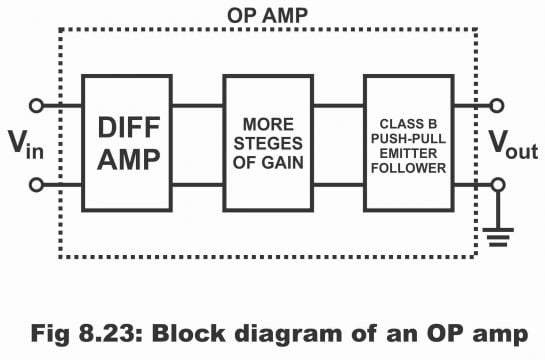Another simple block diagram of an operational amplifier has been displayed. The first stage of it comprises a differential amplifier, (which is used as an input amplifier). After the differential amplifier, 2nd stage occurs which consists of a level shifter (normally has a common emitter stage which also provides voltage gain). The basic purpose of this stage is to ensure that the output dc voltage (when both inputs are grounded) must be zero.  However, making the dc output value zero is not practically possible due to characteristics differences between different devices and resistors (although different resistors and devices are fabricated on the same ICs). For example, if β value of a device is 100, others will be 101. For making output dc voltage zero, a null point adjustment is normally provided on the operational amplifier’s ICs. The third or final stage of an operational amplifier is a common collector (or emitter follower) amplifier stage, which apart from providing current gain also keeps the output resistance of an operational amplifier low, as has been shown in the block diagram. As the input stage of an operational amplifier comprises a differential amplifier, therefore input impedance of an operational amplifier is extremely high (particularly if a differential amplifier is operated on a low voltage level). From the analysis of popular 741 operational amplifiers, it is becoming evident that its input stage operates at about 9.5 µA whereas dc power supply 15 (i.e. VEE= -15 and VCC= +15). Under such a situation, its input resistance is extremely high (i.e. 2MΩ approx.)

Figure 8.24 – the block diagram of an op-amp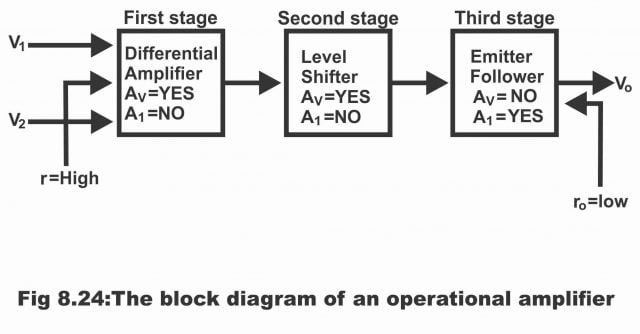## Symbol of OP-AMP

The symbol of an operational amplifier has been demonstrated in figure 8.25. An operational amplifier has at least 5 terminals, out of which two are input terminals, one output terminal and two power supply bias terminals. Names of these terminals have also been written on the symbol as illustrated in the figure.

1). Inverting input terminal

2). Non-inverting input terminal

3). Output terminal (single-ended)

4). Positive bias supply terminal

5). Negative bias supply terminal

In some symbols, positive and negative bias terminals seem juxtaposed whereas in others they seem on one side, as is evident from the triangular type symbol illustrated in the figure.  These are usually standard symbols of an operational amplifier, wherein no change is possible except that negative and positive inputs are sometimes reversed (that’s negative input is made upper while positive input lower occasionally). Sometimes, positive terminal is not revealed at all and it is assumed that it is connected with the ground.

Figure8.26, 8.27– op-amp symbol showing the most important terminalsThe input of an operational amplifier can either be single-ended or double-ended (the double-ended input is called differential input). Single-ended and double-ended differential depends on whether input voltages are going to be provided to one input terminal or to both input terminals. Similarly, amplifiers out can also be either single-ended or double-ended. The most common configuration of an operational amplifier consists of two input terminals and a single-ended output terminal (or a single output terminal).

The symbol of the operational amplifier displayed in the figure reveals that inverting input along with the negative input terminal and non-inverting input along the positive terminal has been emblazoned. It simply means that the signal provided on the negative input terminal would be received in an amplified form on output; however, the phase of this signal on the output terminal will be inverted. Similarly, the signal provided on the positive input terminal will be received in an amplified form on output, however, its phase will not be inverted (i.e. input signal and output signal both will be in-phase or the same). Thus, negative and positive polarity only reveals phase reversal. It does not mean at all that negative voltages have always to be provided on negative or inverting input terminal while positive voltages on non-inverting or positive input terminal. It must also be kept in mind that all input and output voltages are expressed in terms of a common reference (usually ground).

In figure 8.27, another standard symbol of an operational amplifier has been illustrated, in which inverting input (i.e. negative sign) is been made up instead of making it on the lower end, whereas non–inverting input (i.e. positive sign) has been drawn low instead of high side in the diagram (totally opposite to the signs given above). Further, the input signal is denoted by טi. In the majority of applications, one of the amplifier’s inputs is grounded. In such a situation, if an input signal is provided to a non-inverting (positive) terminal, amplifier output becomes in-phase with ט0 input. If the input signal is provided on inverting input terminal (negative terminal), ט0 gets out of phase with respect to the input. This has been elucidated via the table given in the figure.

Figure 8.27 operational amplifier symbol showing inverting (-) and non-inverting (+) inputs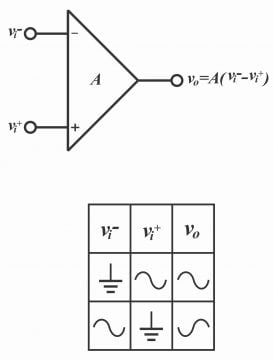## The Ideal Operational Amplifier

We know that an operational amplifier is a direct-coupled amplifier, which has two (differential) inputs and one output. An ideal operational amplifier has the following characteristics.

1). Its open-loop gain tends to be infinite i.e. A ט=∞

2). Its input resistance or impedance (which is measured between the inverting and non-inverting terminals) is infinite (i.e. Ri=Zi=∞)

3). Its output impedance or resistance is zero (i.e. ZO=ZO=0)

4). It has an infinite bandwidth (i.e. BW=∞)

The aforementioned characteristics of an ideal operational amplifier have been shown in the figure 8.28

Figure8.28 – ideal op-amplifier representationAlthough, all the aforementioned characteristics practically do not exist simultaneously in any one amplifier, however, considering an ideal amplifier as a reference, an actual amplifier can be designed almost as closer to it, and the characteristics of a real amplifier can be assessed with respect to the characteristics of an ideal amplifier. The following points of an ideal amplifier must also be instilled in mind.

1). Infinite input resistance or impedance means that the value of input current is zero (I = 0), which means that an ideal operational amplifier is a voltage-controlled device.

2). Zero output resistance or impedance means that Vo does not depend on load resistance parallel to output.

3). Although the gain of an ideal operational amplifier has been assumed as being infinite (Av= ∞), however practically its value is extremely high (approx.106). However, it does not imply that a one-volt signal amplifies to become equal to 106 on output. In fact, the maximum Vo value is limited through bias supply voltage (distinct value of which is (±15v). If  Vo = 15V and Av = 106, the maximum value of input voltage gets limited to 15µV (i.e. /15/106=15µV). Thus, one volt cannot become one million on an operational amplifier; however, 1µV certainly amplifies to 1V. Remember that a large number of modern amplifiers have the capacity of extremely high gain and input impedance while extremely low output impedance.

## The Practical Operational Amplifier

An operational amplifier the gain voltage of which is extremely high but not infinite, the input impedance is extremely high and the output impedance is extremely low, is called a practical operational amplifier. In figure 8.29, a practical operational amplifier has been illustrated. In other words, an operational amplifier consisting of extremely high voltage gain, extremely high impedance, and extremely low output impedance, is known as a practical operational amplifier. That’s main of such an amplifier is neither unlimited nor infinite nor its input impedance is infinity while its output impedance is zero. Its voltage gain, input impedance, and output impedance must have some kind of value.

Figure 8.29 – practical op-amp representationAs every device has some kind of limitations and never possesses characteristics like an ideal amplifier, similarly there are limitations of an integrated operational amplifier as well and one cannot attain characteristics of an ideal amplifier through it. In an operational amplifier, limits on current as well as voltage exist e.g. its peak to peak output voltages are comparatively less than both supply voltages and its output current is also finite due to internal obstacles or prohibitions (e.g. due to power consumption and components ratings). Though characteristics of state-of-the-art modern integrated circuit operational amplifiers are ideal in many respects, however, such a practical amplifier cannot be termed as an ideal nor can it be turned into an ideal device.

## Main Characteristics of OP-AMP

The symbol of a basic operational amplifier is somewhat triangular and normally it has a minimum of five standard connections or terminals (as can be seen from the shape of the symbol in figure 8.25). Often operational amplifiers require bipolar supply (i.e. negative bias supply and positive bias supply). The ground has been connected indirectly with the device through external input and output circuits. The devices which could operate on a single supply, their negative bias supply connections are changed via a ground connection. The output of an operational amplifier is taken from its triangular symbols’ apex. Both inputs are connected with the base manufactured on the opposite side of the triangles’ output. Remember that output polarity always resembles non-inverting input polarity and is always inverse to the inverting input’s polarity (i.e. if inverting polarity is positive with respect to non-inverting polarity, in such a situation output polarity is negative with respect to ground. However, if non-inverting input’s polarity is positive with respect to inverting input, in this case, output polarity is positive with respect to ground)

### Where is Ground

No input of an operational amplifier is connected with the ground (as elucidated via its definition described above). Thus, none of the terminals of an operational amplifier operates as a ground reference. Then where is our ground reference under such a situation? The answer to this question becomes clear via figure 8.30, a reference for all terminal voltages of an operational amplifier. It has been described as follows:

The voltage on operational amplifiers’ positive input with respect to ground = e1

The voltage on operational amplifiers’ negative input with respect to ground =e2

The voltage on output terminal with respect to ground= eout

The voltage on the positive supply terminal with respect to ground= +V1

The voltage on the negative supply terminal with respect to ground= -V2

Figure 8.30 Ground is the common terminal of negative and positive bias supplies. In most circuit schematics, the common ground is not shown, but it is assumed to be at the common terminal of the supplies

figure missing

As the figure shown in diagram (A) is complex and complicated, therefore difficulty is being experienced in reflecting it in the circuit. Thus, the figure is expressed in a simple manner similar to figure (B), in which ground has not been shown. However, it is always assumed that the ground is present on a common terminal of negative and positive supplies. Thus, voltages are expressed or presumed with respect to this unseen ground on different terminals of operational amplifiers. Remember that for simplifying the symbol of an operational amplifier, power supply terminals are not shown on a large number of circuits on its symbol.

### Bias Supply Values

In a majority of amplifiers, symmetrical positive and negative supply voltages are used (i.e. values of both supplies is symmetrical). Most of the common voltage supplies are volts. Through the application of negative and positive bias supplies, output voltages of operational amplifiers keep swinging between positive and negative with respect to the ground. Some of the amplifiers are provided with just a single polarity supply (e.g. 0V – 30V), which is being provided to the supply terminals. Under such a situation, eout polarity remains according to the supply polarity.

### Input and Output Characteristics

The operational amplifier is a differential amplifier which means that it amplifies the difference existing in voltages on the two input terminals. Its output voltage can be expressed through an equation as follows:

E out = A VOL (e1 –e2)

Here, e1 and e2 are voltages on positive and negative input terminals whereas A VOL means amplifiers’ open-loop voltage gain (i.e. voltage gain of an operational amplifier without any external feedback connections). The term (e1 – e2) shows the difference in voltages on input terminals. Often, this voltage difference is also expressed as a differential input ed, as illustrated vide figure 8.31 i.e.

Figure 8.31 Op-AMP amplifies the difference between e1 and e2It is to be remembered that when e1 value exceeds e2, (i.e. e1 > e2), ed will be positive and when value of e1 is less than e2, (i.e. e1<e2), ed will be negative. It must also be kept in mind that an operational amplifier amplifies the differential between e1 and e2 instead of amplifying it themselves. Above eout equation can also be expressed as

eout=AVOL ed

Modern operational amplifiers have extreme values (1000- 100000) of open-loop voltage gain (AVOL). Loop-gain of a 741 operational amplifier is 100,000. It has to be remembered that differential voltage AVOL produces eout via amplifying through ed. When an operational amplifier is used in closed-loop mode, this operation occurs in that situation as well.  The following important points become clear as a result of the above discussion:

i). When ed value is positive, the positive input terminal of the operational amplifier is positive with respect to its negative input terminal and eout is positive.

ii). When ed value is negative, the positive input terminal of operational amplifier is negative with respect to its negative input terminal. In such a situation, eout is negative.

iii). When the positive terminal is grounded (i.e. e2=0) providing voltages to the negative terminal, output polarity becomes inverse relative to the input polarity. That’s the reason negative input is often known as inverting input.

iv). When negative terminal is grounded, (i.e. e2=0) providing voltages to the positive terminal, output and input polarity are same. That’s the reason positive input is often known as non-inverting input.

v). When values of e1 and e2 are mutually equal (i.e. e1=e2), the output is zero.

### Output Voltage Saturation

The maximum output voltage amplitude (the height of a changing quantity is called amplitude) of an operational amplifier can be confined (limited) through the size of power supply voltages provided on operational amplifiers’ bias terminals. The specific value of the maximum amplitude is normally less 1.5 volt compared to the bias voltage supply. For example, if the bias supply voltage value is, the amplitude of eout can be limited to 13.5, which is represented by drawing a graph between eout and ed, as has been illustrated in figure 8.32.

Figrue 8.32 missing

Here the value open-loop gain voltage value of the operational amplifier has been given as 10,000.

The graph reveals that eout change linearly up to a very small range of ed (i.e. they change together). When ed value increases beyond a certain limit, eout saturate on 13.5 volt or 13.5 volt. This kind of saturation on the polarity of ed. The value of ed, on which saturation occurs, has been demonstrated through following formula:

ed = +13.5V/AVOL=  /10000= 1.35mV

It shows that output saturates by providing 1.35 volt of any polarity parallel to the input terminals. The open-loop gain in a majority of operational amplifiers is even higher than 100,000. Saturation is produced by providing just 35µv of any polarity of this value on the differential input of open-loop gain. It means that due to the very minute differential in the voltages provided on the operational amplifiers’ input, it will operate in its linear range. However, differential ed is kept very low for linear operators in a number of operational amplifiers by using negative feedback in its circuit. Remember that when an operational amplifier is in its linear range, (i.e. does not saturate) the ed value is very low. That’s in such a situation, voltages found parallel to input terminals are considered zero.

Thus, characteristics of an operation amplifier are as below:

1). It is a DC (direct current) amplifier

2). Its voltage gain (in an ideal situation) is very high

3). Its input impedance is very high (in a typical situation)

4). Its output impedance is very low (in an ideal situation)

5). When its input is at zero volts, its output is also zero.

6). Its output can be changed between both extremely positive and negative voltage values i.e. it swings between positive and negative.

7). Its common mode rejection ratio (CMRR) is extremely high (it means that it rejects unpleasant noise and hum signals quite effectively)

8). It operates with single-ended output and differential inputs (though inputs are mostly grounded)

9). If an operational amplifier is operated via a dual power supply (VCC and VFF), it can then amplify both the halves of input AC signal. The specific values of a dual power supply are  15V

10). Its output polarity is always similar to non- inverting input polarity and always inverse to an inverting input polarity.

## Equivalent Circuit of OP-AMP

An operational amplifier can also be revealed via an ordinary equivalent circuit just like all other voltage amplifiers. In figure 8.33, equivalent circuit parallel to an operational amplifier has been demonstrated. The input side has been shown via an impedance Zin (or resistance Rin), which is the differential input (or resistance) of an operational amplifier i.e. resistance or impedance between input terminals. Output side reflected via a dependent source the amplitude of which is A VOL x ed (or AOL (V1-V2)) and an output impedance Zout (or an output resistance Rout) This equivalent circuit is acceptable only in the linear region. Remember that input resistance or impedance values of an operational amplifier are normally very high (10 ohm- 1 mega ohm). The input impedance of all popular 741 operational amplifiers is up to 2MΩ, while value of its output impedance normally ranges between 50Ω to 200MΩ,

which is very small relative to its input impedance. (The specific output value of a 741 operational amplifier is up to75Ω). However, when the operational amplifier is used with negative feedback, its effective output impedance reduces from 1 ohm. In such a situation, this amplifier drives maximum loads with minimal amplitude loss.

Figure 8.33 (a) Op-Amp equivalent circuit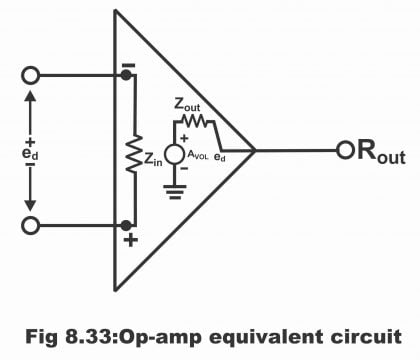An operational amplifier’s maximum output current’s specific value tends to be within a range from 5mA to 25mA. Relatively a small output current is not considered a drawback because, in several applications of operational amplifiers, its output keeps driving some other operational circuit along with its high input impedance. When a high current load is desired to be driven, a high current transistor is applied for amplifying the output current of an operational amplifier.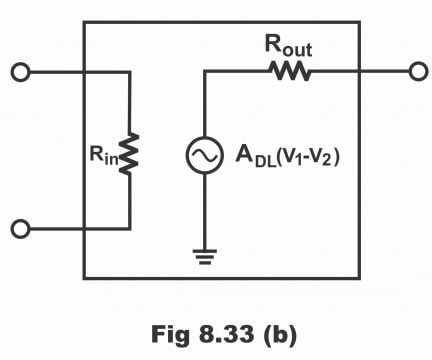## Working Method of a Common OP-AMP

An operational amplifier basically is a directly coupled high gain amplifier, through the feedback of which, its collective response characteristics are controlled. In its integrated form, a completed amplifier circuit is assembled on a small silicon chip and is enclosed in a capsule. Pins are fitted on suitable amplifier spots which have been jutted out of the capsule. For using amplifiers, these pins are properly fitted along with the other external components, thus, a specific response is received from an operational amplifier.

An operational amplifier consists of several stages fitted on a cascade. Every amplifier stage keeps on providing some adorable or pleasant characteristics to the completed circuit and due to these specific characteristics; an operational amplifier can be distinguished from an ordinary amplifier.

In figure 8.34, a block diagram of a particular operational amplifier has been displayed. An operational amplifier usually is a collection of three stages (as displayed in the figure). Every stage provides some top-quality characteristics (i.e. every stage has certain peculiar characteristics)

Figure 8.34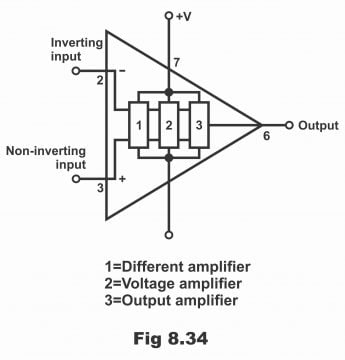The input stage of an operational amplifier consists of a differential amplifier, which offers several advantages to an operational amplifier i.e. high common-mode rejection, differential inputs, and provision of frequency response to DC etc. Besides, some specific techniques are also applied in the input stage in order to enhance the input impedance of the input stage (in other words, a differential amplifier is fitted on the input stage of an operational amplifier, due to which common-mode signals on the operation amplifier come to an end. Differential inputs are received and frequency response gets to a very low frequency or accurate dc. Besides, special kinds of techniques are used for providing high impedance to the input stage)

The second stage comprises a high gain voltage amplifier. This stage is a collection of a number of transistors which are usually configured as Darlington Pairs. The gain of this specific type of an operational amplifier is about 200,000 or even higher. Thus, the stage generates a major gain share.

The third stage comprises an output amplifier. This stage is normally in the complementary emitter-follower shape. The stage provides low output impedance to the operational amplifier, resultantly operational amplifier provides several Mille amperes of current to the load.

The operational amplifier is provided a dual power supply (i.e. both [positive as well as a negative type of power supply), due to which output voltages are sometimes either positive or negative with respect to the ground (i.e. swing between positive and negative).

The circuit diagram of an accomplished operational amplifier has been demonstrated in figure 8.35. In this circuit, three stages have also separately been shown. No coupling capacitors have been used in this circuit at all rather; transistors have mostly been used. This operational amplifier can amplify DC signals as well along with AC signals. Two terminals i.e. 1 -5 in the operational amplifier block diagram are such that they have not been revealed on the circuit. Their name is “offset null”. The external potentiometer is connected with a circuit through the terminals. The potentiometer has been set in such a manner that when both inputs of an operational amplifier are zero, the output of the operational amplifier also becomes exactly zero. A positive (+ive) and negative (-ive) sign has been made on the terminals. Negative input is called inverting input whereas positive input is known as non-inverting input.

One of the inputs of an operational amplifier has been grounded for being used as an amplifier. The signal which is meant to be amplified is provided on other input. If positive input is grounded and signal provided on negative input, in such a situation output is inverted to input, however, it is received in an amplified form. On the contrary, if negative input is grounded and signals and signals provided on positive input, the signal amplifies but it is not inverted to input (I.e. non- inverted but amplified output is received). Both input terminals are connected directly on Q1 and Q2 base.

Figure 8.35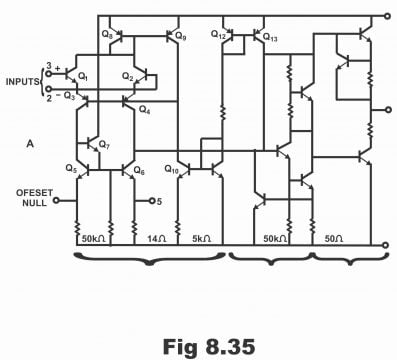It must be remembered that there is no base resistor in an operational amplifier. Similarly, DC current repulses back to the ground via an external circuit. Normally, it is not considered an issue as DC is grounded via a signal source. However, in some of the situations, the coupling is also done within different stages through capacitors. For this purpose, a resistor is added for providing a retreating route to DC. The standard symbol of an operational amplifier resembles a triangle as shown in figure 8.30. Supply connections have been reflected in figure (A), whereas these supply connections have not been revealed in symbol figure (B). For simplicity purposes, supply terminals have not been depicted. However, it has to be kept in mind that supply terminals do have a presence. In figure 8.36, standard packages of an operational amplifier have also been shown.

Figure 8.36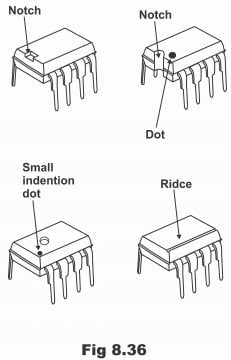Next Topic: Different Types of OP AMP Parameters

Previous Topic: IC Package

For electronics and programming-related projects visit my YouTube channel.